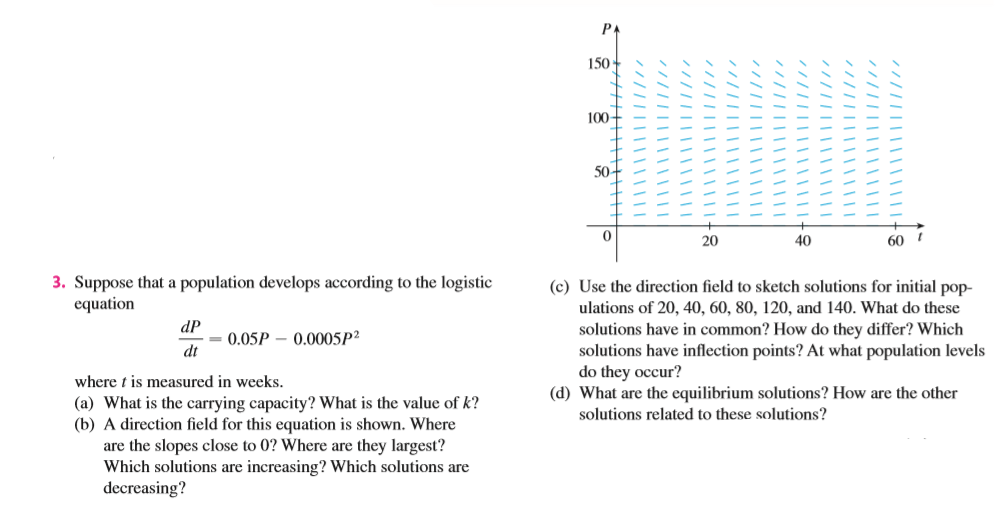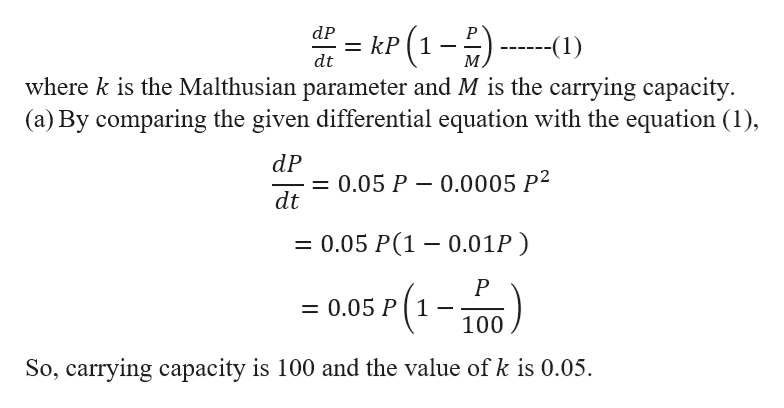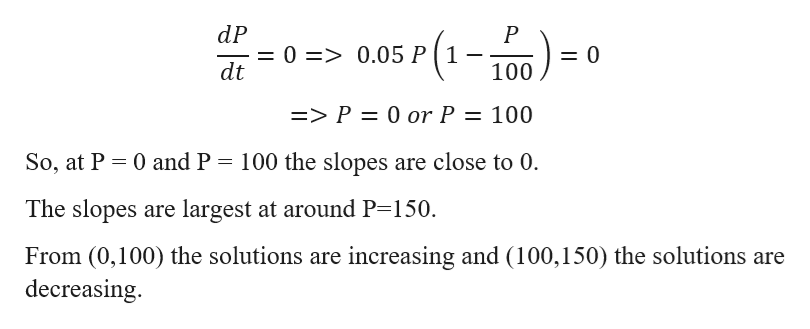# 150100502040603. Suppose that a population develops according to the logisticequation(c) Use the direction field to sketch solutions for initial pop-ulations of 20, 40, 60, 80, 120, and 140. What do thesedP= 0.05P - 0.0005P2dtsolutions have in common? How do they differ? Whichsolutions have inflection points? At what population levelsdo they occur?(d) What are the equilibrium solutions? How are the othersolutions related to these solutions?where t is measured in weeks.(a) What is the carrying capacity? What is the value of k?(b) A direction field for this equation is shown. Whereare the slopes close to 0? Where are they largest?Which solutions are increasing? Which solutions aredecreasing?

Question
204 viewshelp_outlineImage Transcriptionclose150 100 50 20 40 60 3. Suppose that a population develops according to the logistic equation (c) Use the direction field to sketch solutions for initial pop- ulations of 20, 40, 60, 80, 120, and 140. What do these dP = 0.05P - 0.0005P2 dt solutions have in common? How do they differ? Which solutions have inflection points? At what population levels do they occur? (d) What are the equilibrium solutions? How are the other solutions related to these solutions? where t is measured in weeks. (a) What is the carrying capacity? What is the value of k? (b) A direction field for this equation is shown. Where are the slopes close to 0? Where are they largest? Which solutions are increasing? Which solutions are decreasing? fullscreen
check_circle

Step 1

Given that a population develops according to the logistic equation

Step 2

We know the Logistic Model equation ishelp_outlineImage TranscriptioncloseP(1-) where k is the Malthusian parameter and M is the carrying capacity (a) By comparing the given differential equation with the equation (1) dP kP -(1) M dt dP = 0.05 P dt 0.0005 P2 = 0.05 P(1 0.01P) P = 0.05 P 1 100 So, carrying capacity is 100 and the value of k is 0.05 fullscreen
Step 3

Now,

...help_outlineImage TranscriptionclosedP = 0 => 0.05 P ( 1 - dt sr(1-100) P = 0 => P = 0 or P 100 11 So, at P 0 and P 100 the slopes are close to 0. The slopes are largest at around P=150. From (0,100) the solutions are increasing and (100,150) the solutions are decreasing fullscreen

### Want to see the full answer?

See Solution

#### Want to see this answer and more?

Solutions are written by subject experts who are available 24/7. Questions are typically answered within 1 hour.*

See Solution
*Response times may vary by subject and question.
Tagged in

### Integration